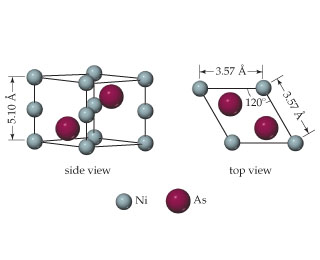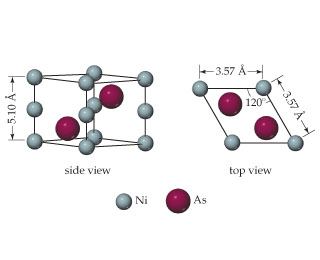# Problem: The unit cell of nickel arsenide is shown here. What is the empirical formula?

###### FREE Expert Solution

We are asked the empirical formula of Nickel Arsenide from the Unit cell given.

Nickel Arsenide follows an hcp or hexagonal closed packed unit cell which is composed of a cube with one eight atoms at each of its corners, four atoms at its edges and two atoms at the center of the cube.83% (492 ratings)###### Problem Details

The unit cell of nickel arsenide is shown here.What is the empirical formula?